## 10.3 Conditions for inference for regression

Recall in Subsection 8.3.2 we stated that we could only use the standard-error-based method for constructing confidence intervals if the bootstrap distribution was bell shaped. Similarly, there are certain conditions that need to be met in order for the results of our hypothesis tests and confidence intervals we described in Section 10.2 to have valid meaning. These conditions must be met for the assumed underlying mathematical and probability theory to hold true.

For inference for regression, there are four conditions that need to be met. Note the first four letters of these conditions are highlighted in bold in what follows: LINE. This can serve as a nice reminder of what to check for whenever you perform linear regression.

1. Linearity of relationship between variables
2. Independence of the residuals
3. Normality of the residuals
4. Equality of variance of the residuals

Conditions L, N, and E can be verified through what is known as a residual analysis. Condition I can only be verified through an understanding of how the data was collected.

In this section, we’ll go over a refresher on residuals, verify whether each of the four LINE conditions hold true, and then discuss the implications.

### 10.3.1 Residuals refresher

Recall our definition of a residual from Subsection 5.1.3: it is the observed value minus the fitted value denoted by $$y - \widehat{y}$$. Recall that residuals can be thought of as the error or the “lack-of-fit” between the observed value $$y$$ and the fitted value $$\widehat{y}$$ on the regression line in Figure 10.1. In Figure 10.2, we illustrate one particular residual out of 463 using an arrow, as well as its corresponding observed and fitted values using a circle and a square, respectively.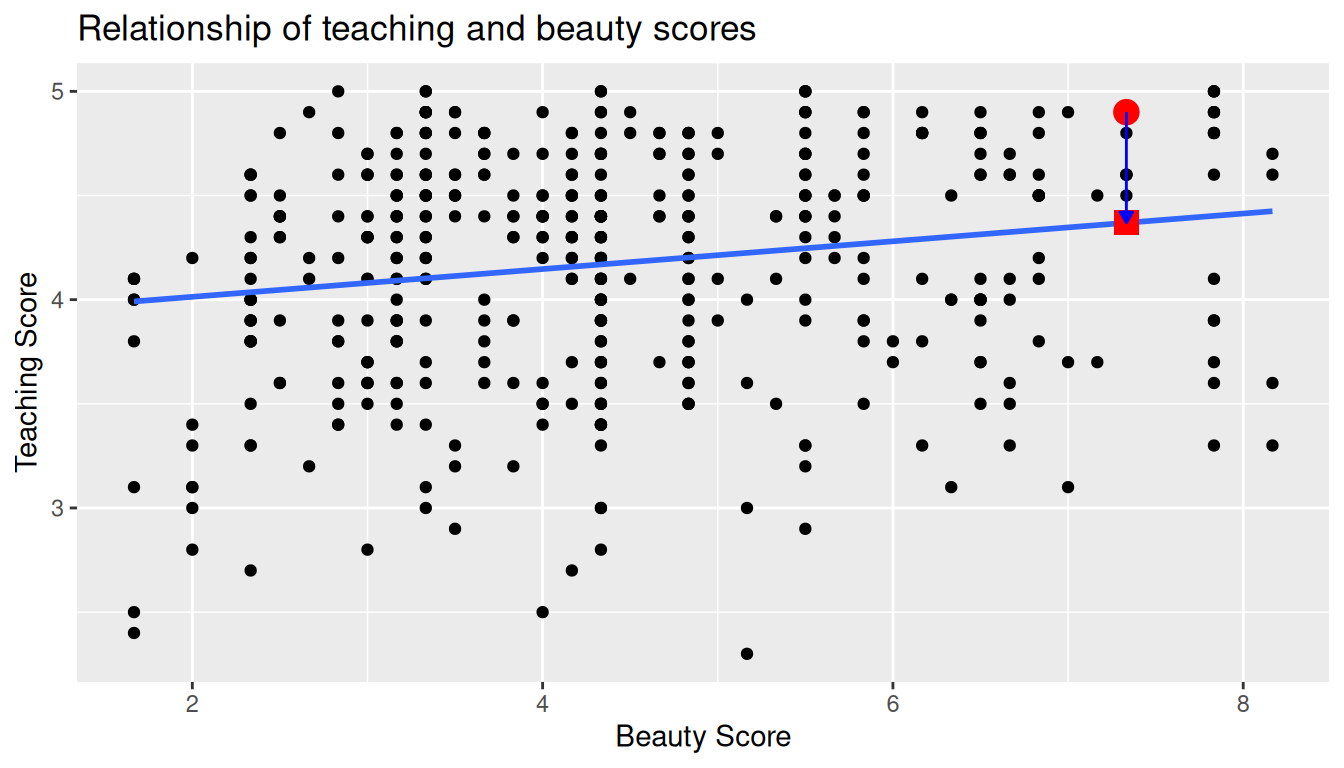FIGURE 10.2: Example of observed value, fitted value, and residual.

Furthermore, we can automate the calculation of all $$n$$ = 463 residuals by applying the get_regression_points() function to our saved regression model in score_model. Observe how the resulting values of residual are roughly equal to score - score_hat (there is potentially a slight difference due to rounding error).

# Fit regression model:
score_model <- lm(score ~ bty_avg, data = evals_ch5)
# Get regression points:
regression_points <- get_regression_points(score_model)
regression_points
# A tibble: 463 x 5
ID score bty_avg score_hat residual
<int> <dbl>   <dbl>     <dbl>    <dbl>
1     1 4.7   5           4.214  0.486
2     2 4.100 5           4.214 -0.114
3     3 3.9   5           4.214 -0.314
4     4 4.8   5           4.214  0.586
5     5 4.600 3           4.08   0.52
6     6 4.3   3           4.08   0.22
7     7 2.8   3           4.08  -1.28
8     8 4.100 3.333       4.102 -0.002
9     9 3.4   3.333       4.102 -0.702
10    10 4.5   3.16700     4.091  0.40900
# … with 453 more rows

A residual analysis is used to verify conditions L, N, and E and can be performed using appropriate data visualizations. While there are more sophisticated statistical approaches that can also be done, we’ll focus on the much simpler approach of looking at plots.

### 10.3.2 Linearity of relationship

The first condition is that the relationship between the outcome variable $$y$$ and the explanatory variable $$x$$ must be Linear. Recall the scatterplot in Figure 10.1 where we had the explanatory variable $$x$$ as “beauty” score and the outcome variable $$y$$ as teaching score. Would you say that the relationship between $$x$$ and $$y$$ is linear? It’s hard to say because of the scatter of the points about the line. In the authors’ opinions, we feel this relationship is “linear enough.”

Let’s present an example where the relationship between $$x$$ and $$y$$ is clearly not linear in Figure 10.3. In this case, the points clearly do not form a line, but rather a U-shaped polynomial curve. In this case, any results from an inference for regression would not be valid.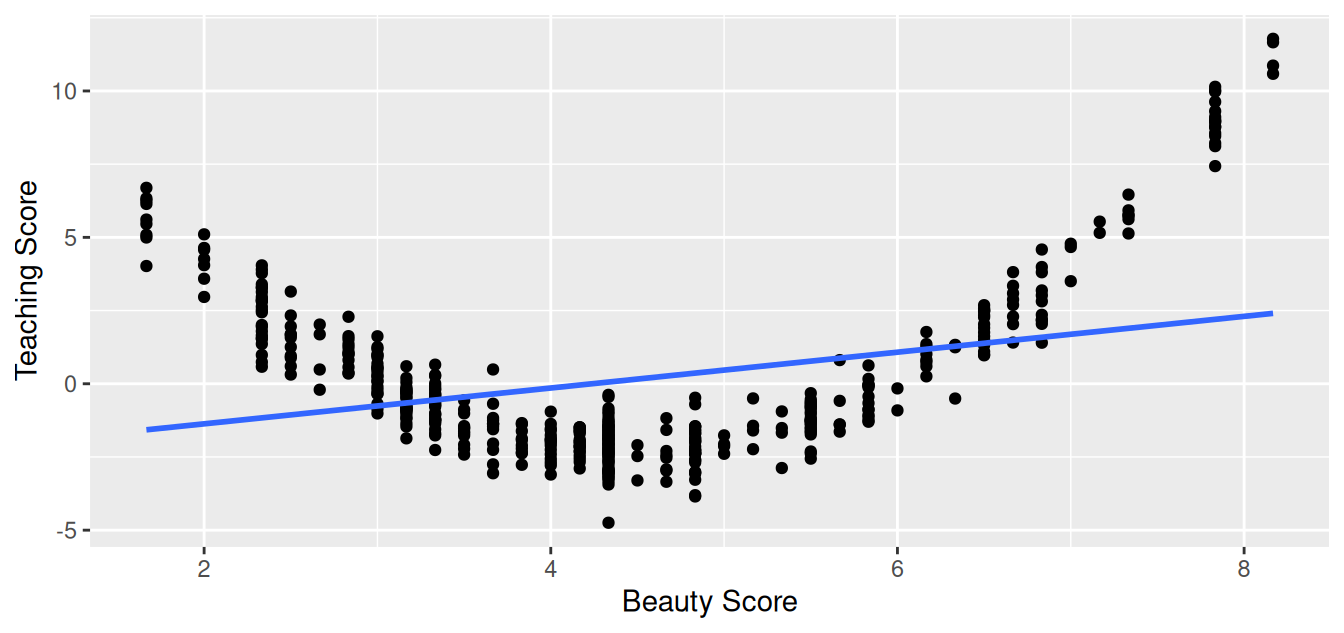FIGURE 10.3: Example of a clearly non-linear relationship.

### 10.3.3 Independence of residuals

The second condition is that the residuals must be Independent. In other words, the different observations in our data must be independent of one another.

For our UT Austin data, while there is data on 463 courses, these 463 courses were actually taught by 94 unique instructors. In other words, the same professor is often included more than once in our data. The original evals data frame that we used to construct the evals_ch5 data frame has a variable prof_ID, which is an anonymized identification variable for the professor:

evals %>%
select(ID, prof_ID, score, bty_avg)
# A tibble: 463 x 4
ID prof_ID score bty_avg
<int>   <int> <dbl>   <dbl>
1     1       1 4.7   5
2     2       1 4.100 5
3     3       1 3.9   5
4     4       1 4.8   5
5     5       2 4.600 3
6     6       2 4.3   3
7     7       2 2.8   3
8     8       3 4.100 3.333
9     9       3 3.4   3.333
10    10       4 4.5   3.16700
# … with 453 more rows

For example, the professor with prof_ID equal to 1 taught the first 4 courses in the data, the professor with prof_ID equal to 2 taught the next 3, and so on. Given that the same professor taught these first four courses, it is reasonable to expect that these four teaching scores are related to each other. If a professor gets a high score in one class, chances are fairly good they’ll get a high score in another. This dataset thus provides different information than if we had 463 unique instructors teaching the 463 courses.

In this case, we say there exists dependence between observations. The first four courses taught by professor 1 are dependent, the next 3 courses taught by professor 2 are related, and so on. Any proper analysis of this data needs to take into account that we have repeated measures for the same profs.

So in this case, the independence condition is not met. What does this mean for our analysis? We’ll address this in Subsection 10.3.6 coming up, after we check the remaining two conditions.

### 10.3.4 Normality of residuals

The third condition is that the residuals should follow a Normal distribution. Furthermore, the center of this distribution should be 0. In other words, sometimes the regression model will make positive errors: $$y - \widehat{y} > 0$$. Other times, the regression model will make equally negative errors: $$y - \widehat{y} < 0$$. However, on average the errors should equal 0 and their shape should be similar to that of a bell.

The simplest way to check the normality of the residuals is to look at a histogram, which we visualize in Figure 10.4.

ggplot(regression_points, aes(x = residual)) +
geom_histogram(binwidth = 0.25, color = "white") +
labs(x = "Residual")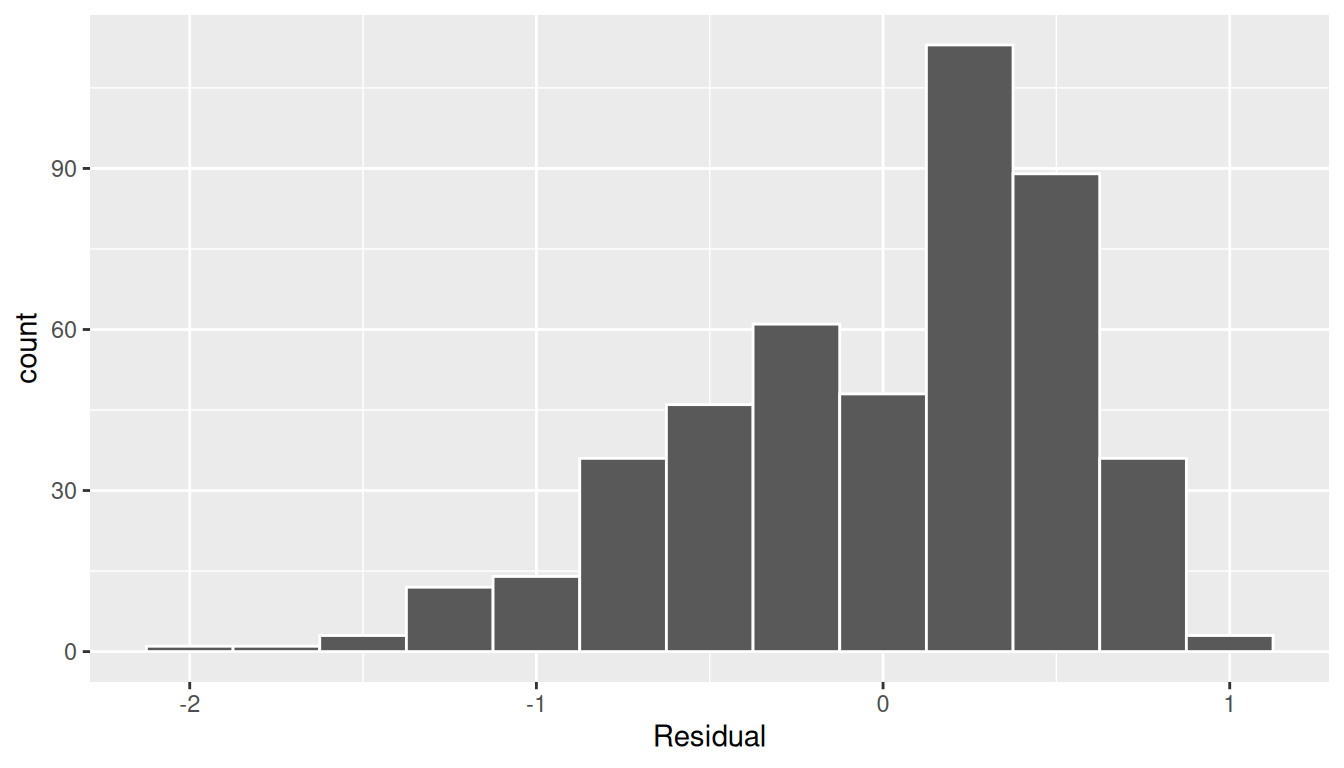FIGURE 10.4: Histogram of residuals.

This histogram shows that we have more positive residuals than negative. Since the residual $$y-\widehat{y}$$ is positive when $$y > \widehat{y}$$, it seems our regression model’s fitted teaching scores $$\widehat{y}$$ tend to underestimate the true teaching scores $$y$$. Furthermore, this histogram has a slight left-skew in that there is a tail on the left. This is another way to say the residuals exhibit a negative skew.

Is this a problem? Again, there is a certain amount of subjectivity in the response. In the authors’ opinion, while there is a slight skew to the residuals, we feel it isn’t drastic. On the other hand, others might disagree with our assessment.

Let’s present examples where the residuals clearly do and don’t follow a normal distribution in Figure 10.5. In this case of the model yielding the clearly non-normal residuals on the right, any results from an inference for regression would not be valid.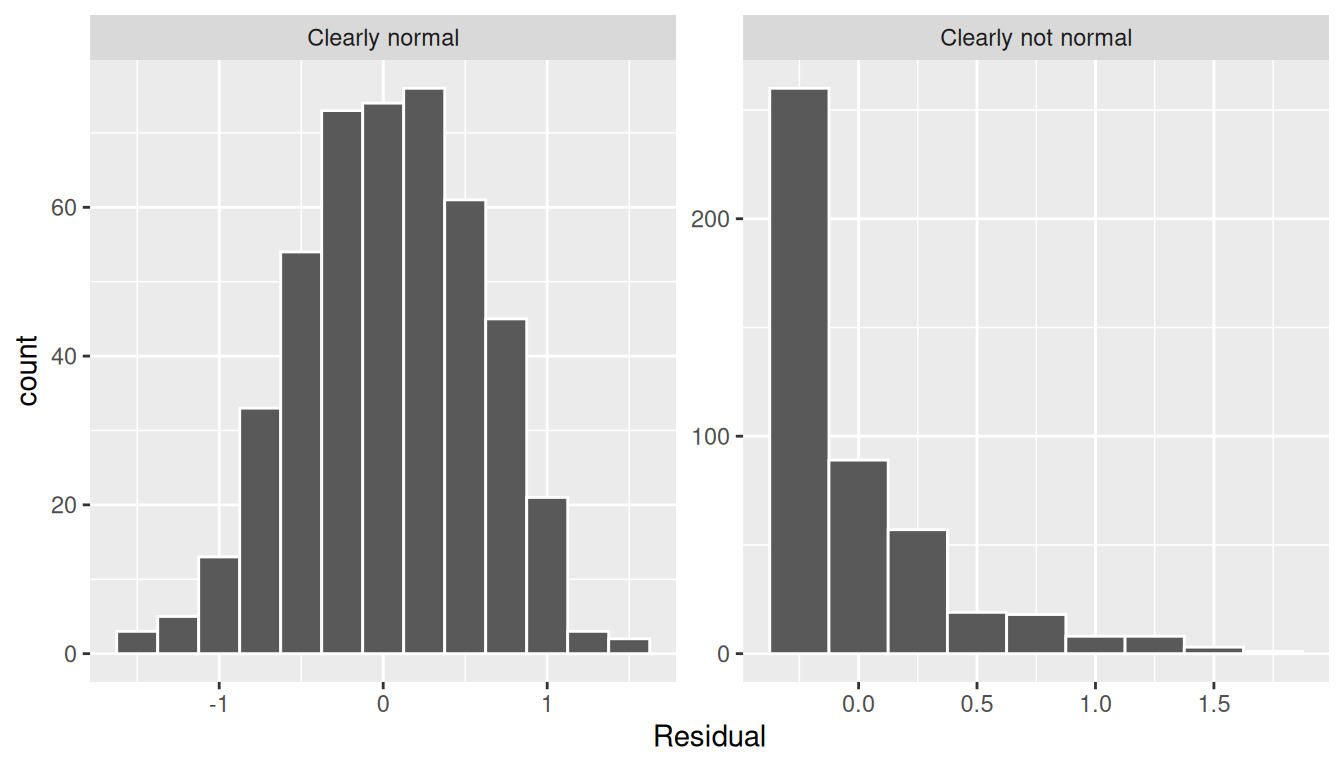FIGURE 10.5: Example of clearly normal and clearly not normal residuals.

### 10.3.5 Equality of variance

The fourth and final condition is that the residuals should exhibit Equal variance across all values of the explanatory variable $$x$$. In other words, the value and spread of the residuals should not depend on the value of the explanatory variable $$x$$.

Recall the scatterplot in Figure 10.1: we had the explanatory variable $$x$$ of “beauty” score on the x-axis and the outcome variable $$y$$ of teaching score on the y-axis. Instead, let’s create a scatterplot that has the same values on the x-axis, but now with the residual $$y-\widehat{y}$$ on the y-axis as seen in Figure 10.6.

ggplot(regression_points, aes(x = bty_avg, y = residual)) +
geom_point() +
labs(x = "Beauty Score", y = "Residual") +
geom_hline(yintercept = 0, col = "blue", size = 1)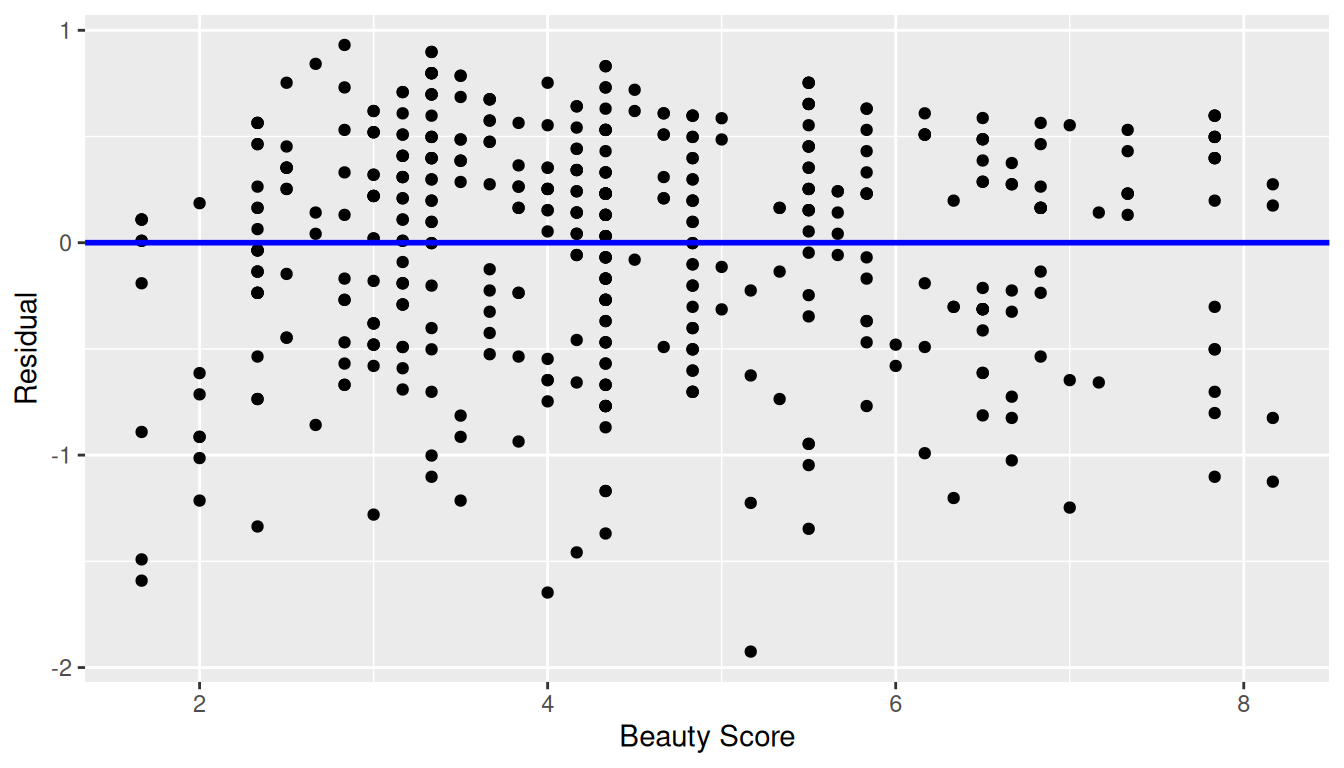FIGURE 10.6: Plot of residuals over beauty score.

You can think of Figure 10.6 as a modified version of the plot with the regression line in Figure 10.1, but with the regression line flattened out to $$y=0$$. Looking at this plot, would you say that the spread of the residuals around the line at $$y=0$$ is constant across all values of the explanatory variable $$x$$ of “beauty” score? This question is rather qualitative and subjective in nature, thus different people may respond with different answers. For example, some people might say that there is slightly more variation in the residuals for smaller values of $$x$$ than for higher ones. However, it can be argued that there isn’t a drastic non-constancy.

In Figure 10.7 let’s present an example where the residuals clearly do not have equal variance across all values of the explanatory variable $$x$$.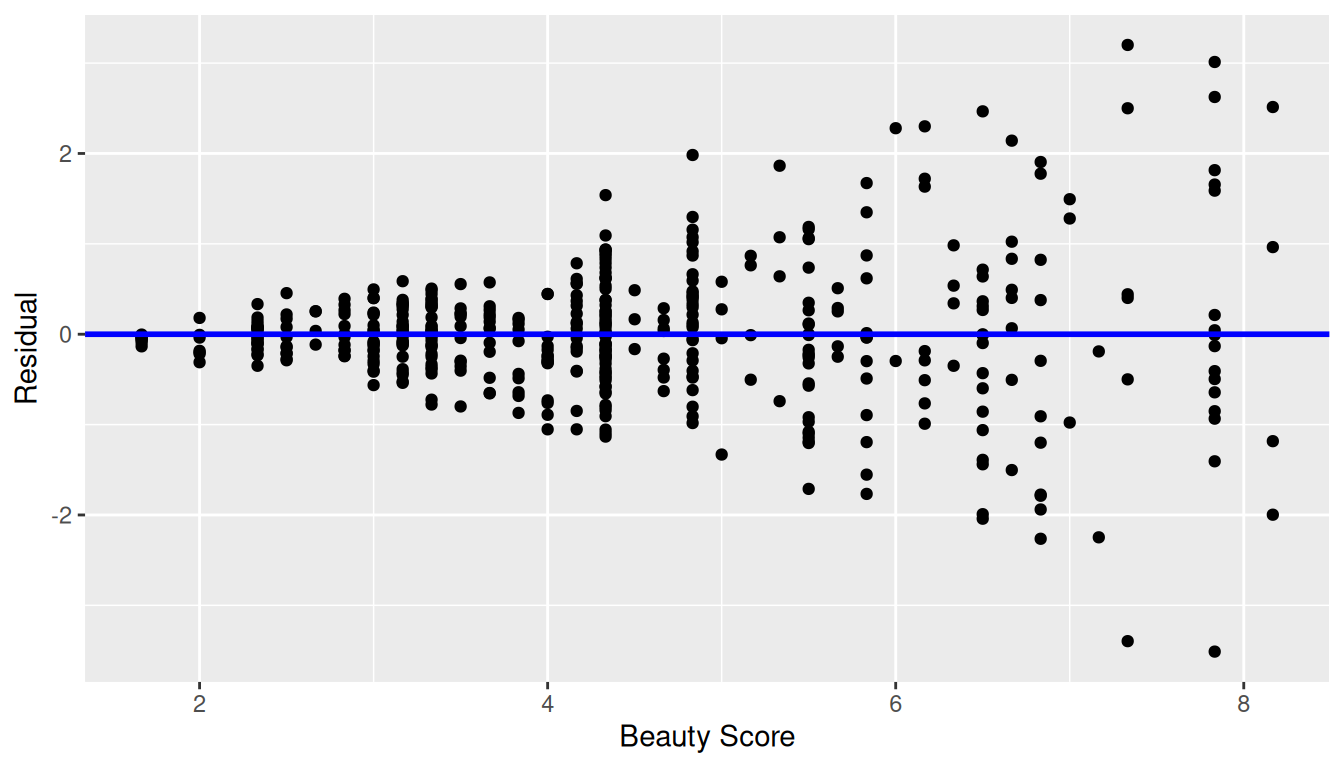FIGURE 10.7: Example of clearly non-equal variance.

Observe how the spread of the residuals increases as the value of $$x$$ increases. This is a situation known as heteroskedasticity. Any inference for regression based on a model yielding such a pattern in the residuals would not be valid.

### 10.3.6 What’s the conclusion?

Let’s list our four conditions for inference for regression again and indicate whether or not they were satisfied in our analysis:

1. Linearity of relationship between variables: Yes
2. Independence of residuals: No
3. Normality of residuals: Somewhat
4. Equality of variance: Yes

So what does this mean for the results of our confidence intervals and hypothesis tests in Section 10.2?

First, the Independence condition. The fact that there exist dependencies between different rows in evals_ch5 must be addressed. In more advanced statistics courses, you’ll learn how to incorporate such dependencies into your regression models. One such technique is called hierarchical/multilevel modeling.

Second, when conditions L, N, E are not met, it often means there is a shortcoming in our model. For example, it may be the case that using only a single explanatory variable is insufficient, as we did with “beauty” score. We may need to incorporate more explanatory variables in a multiple regression model as we did in Chapter 6.

In our case, the best we can do is view the results suggested by our confidence intervals and hypothesis tests as preliminary. While a preliminary analysis suggests that there is a significant relationship between teaching and “beauty” scores, further investigation is warranted; in particular, by improving the preliminary score ~ bty_avg model so that the four conditions are met. When the four conditions are roughly met, then we can put more faith into our confidence intervals and $$p$$-values.

The conditions for inference in regression problems are a key part of regression analysis that are of vital importance to the processes of constructing confidence intervals and conducting hypothesis tests. However, it is often the case with regression analysis in the real world that not all the conditions are completely met. Furthermore, as you saw, there is a level of subjectivity in the residual analyses to verify the L, N, and E conditions. So what can you do? We as authors advocate for transparency in communicating all results. This lets the stakeholders of any analysis know about a model’s shortcomings or whether the model is “good enough.” So while this checking of assumptions has lead to some fuzzy “it depends” results, we decided as authors to show you these scenarios to help prepare you for difficult statistical decisions you may need to make down the road.

Learning check

(LC10.1) Continuing with our regression using age as the explanatory variable and teaching score as the outcome variable.

• Use the get_regression_points() function to get the observed values, fitted values, and residuals for all 463 instructors.
• Perform a residual analysis and look for any systematic patterns in the residuals. Ideally, there should be little to no pattern but comment on what you find here.